# KCET 2018 Physics Paper with Solutions

KCET 2018 Physics paper is the best resource to gain insight into the examination. KCET 2018 exam conducted by KEA is one of the most sought out examinations by the students of Karnataka who are aspiring to get admission in prestigious institutions. The solutions uploaded at BYJU’S will enable the students to divide and plan their resources in an optimal way. Practising these questions will help the students to achieve their goals. Solving these papers will help the candidates in understanding the questions paper pattern, time management and also help them identify their strengths and weaknesses.

### KCET 2018 - Physics

Question 1: A particle shows the distance-time curve as shown in the figure. The maximum instantaneous velocity of the particle is around the point.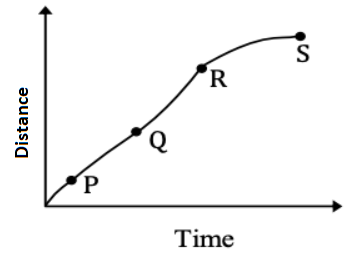1. a. P
2. b. S
3. c. R
4. d. Q

Solution:

Vm ax = dx/dt = maximum slope

From the figure, at point R the slope is maximum, hence at this point velocity is maximum.

∴ The maximum instantaneous velocity of the particle is around the point ‘R’.

Question 2: Which of the following graphs correctly represents the variation of ‘g’ on the Earth?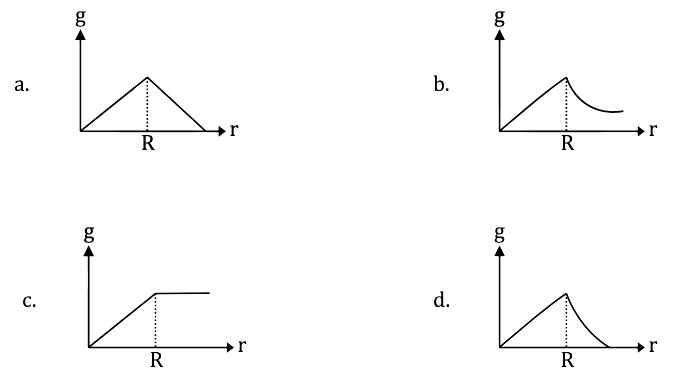Solution: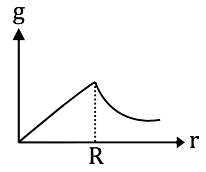For an isothermal process,

B = [1 / P] c

The graph will be a rectangular hyperbola.

Question 3: A cup of tea cools from 65.5°C to 62.5°C in 1 minute in a room at 22.5°C. How long will it take to cool from 46.5°C to 40.5°C in the same room?

1. a. 4 minutes
2. b. 2 minutes
3. c. 1 minute
4. d. 3 minutes

Solution:

T1 = 65.5°C, T2 = 62.5°C

Room temperature (T0) = 22.5°C

Ist Case:-

Using Newton’s law cooling,

[T1 - T2] / t = –k [{[T1 – T2] / t} - T0]

[65.5 – 62.5] / 1 = –k [{[65.5 – 62.5] / 1} - 22.5]

3 = – k [64 – 22.5]

3 = – k [41.5] ------- (1)

IInd Case:-

T1 = 46.5°C, T2 = 40.5°C

[46.5 – 40.5] / t = –k [{[46.5 + 40.5] / 2} - 22.5]

(6 / t) = – k [43.5 – 22.5]

(6 / t) = – k  ---- (2)

Equation (1) dividing by Equation (2),

3 / [6 / t] = – k [41.5] / – k 

(t / 2) = 41.5 / 21

After solving, t = 4 min.

Question 4: The dimensions of the ratio of magnetic flux (φ) and permeability (μ) are

1. a. [M0L1T0A1]
2. b. [M0L–3T0A1]
3. c. [M0L1T1A–1]
4. d. [M0L2T0A1]

Solution:

Magnetic flux (φ) = BA

Permeability (μ) = B / H

φ = B.A × μ0 (1 / d) A ⇒ 0 / μ = IA / d

⇒ IL ⇒ [M0L1T0A1]

Question 5: A mass ‘m’ on the surface of the Earth is shifted to a target equal to the radius of the Earth. If ‘R’ is the radius and ‘M’ is the mass of the Earth, then work done in this process is

1. a. mgR / 2
2. b. mgR
3. c. 2 mgR
4. d. mgR / 4

Solution: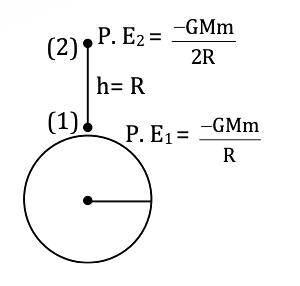P.E. of the earth surface (P.E1) = − GMm / R

P.E. of h height on the surface of earth

P.E,2 = − GNM / [R + R] ⇒ – GMm / 2R

∴ W = Uf – Vi ⇒ P.E.2 – P.E.1 ⇒ {– GMm / 2R} – {− GMm / R}

After solving,

W = –MgR / 2

Question 6: First overtone frequency of a closed pipe of length ‘l1’ is equal to the 2nd harmonic frequency of an open pipe of length ‘l2’. The ratio l1 / l2 =

1. a. 3 / 4
2. b. 4 / 3
3. c. 3 / 2
4. d. 2 / 3

Solution:

First overtone frequency for closed

Organ pipe f = 3V / 4L1

Frequency for open pipe f = 2u / 2L2

⇒ L1 / L2 = 3 / 4

Question 7: The resistance R = V / I where V = (100 ± 5) V and I = (10 ± 0.2) A. The percentage error in R is

1. a. 5.2%
2. b. 4.8%
3. c. 7%
4. d. 3%

Solution:

R = V / I

V = (100 ± 5) V and I = (10 ± 0.2) A

R = 100 / 10 = 10

ΔR / R = ± [(ΔV / V) + (ΔI / I)]

[ΔR / R] * 100 = ± [(ΔV / V) * 100 + (ΔI / I) * 100]

= ± [(5 / 100) * 100 + (0.2 / 10) * 100]

R = ± 7 %

Question 8: A block rests on a rough inclined plane making an angle of 30° with the horizontal. The coefficient of static friction between the block and the plane is 0.8. If the frictional force on the block is 10 N, the mass of the block is (g = 10 ms–2)

1. a. 1 kg
2. b. 2 kg
3. c. 3 kg
4. d. 4 kg

Solution:

N = mg cosθ

Mg sin θ = fs

M × 10 × sin 30 = 10

∴ m = 2 kg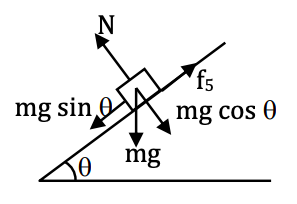Question 9: Two particles of masses m1 and m2 have equal kinetic energies. The ratio of their moments is

1. a. m1 : m2
2. b. m2 : m1
3. c. √m1 : √m2
4. d. m12 : m22

Solution:

Kinetic energy, k = P2 / 2M

∴ P2 = 2 mK

P = √2 mK

P1 / P2 = √[2m1K / 2m2K]

= √m1 / m2

∴ P1 : P2 = √m1 : √m2

Question 10: The pressure at the bottom of a liquid tank is not proportional to the

1. a. Acceleration due to gravity
2. b. The density of the liquid
3. c. Height of the liquid
4. d. Area of the liquid surface

Solution:

The pressure depends on acceleration, the height of the liquid and the density of the liquid. Pressure does not depend on the area of the liquid surface.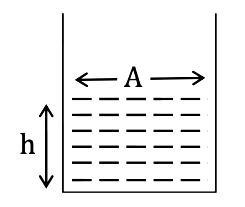Force (F) = (h . A) ρ.g

F = hAρg

Pressure (P) = F / A

P = hρg

Question 11: A Carnot engine takes 300 calories of heat from a source at 500 K and rejects 150 calories of heat to the sink. The temperature of the sink is

1. a. 125 K
2. b. 250 K
3. c. 750 K
4. d. 1000 K

Solution:

Given:- T1 = 500 k

Q1 = 300 calories

Q3 = 150 calories

T2 = ?

Q2 / Q1 = T2 / T1

T2 = [Q2 × T1] / Q1

T2 = [150 × 500] / 300

T2 = 250 K

Question 12: Pressure of an ideal gas is increased by keeping the temperature constant. The kinetic energy of molecules

1. a. Decreases
2. b. Increases
3. c. Remains the same
4. d. Increases or decreases depending on the nature of gas

Solution:

PV = nRT

Hence P ∝ V

Temperature is constant and there is no change in Kinetic energy of molecules.

Question 13: A man weighing 60 kg is in a lift moving down with an acceleration of 1.8 ms–2. The force exerted by the floor on him is

1. a. 588 N
2. b. 480 N
3. c. Zero
4. d. 696 N

Solution: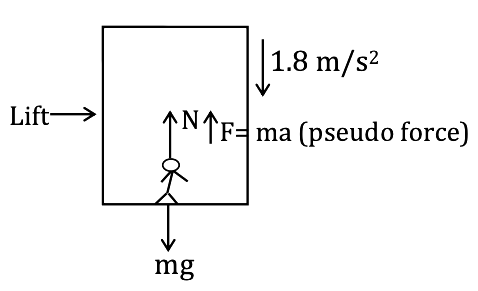Lift –

Now –

N + F = mg

[Where F is a Pseudo force]

N = mg – F

= mg – ma

= m (g – a)

= 60 (9.8 – 1.8)

= 60 × 8 N

= 480 N

Question 14: Moment of inertia of a body about two perpendicular axes X and Y in the plane of the lamina are 20 kg m2 respectively. Its moment of inertia about an axis perpendicular to the plane of the lamina and passing through the point of intersection of X and Y axes is

1. a. 5 kg m2
2. b. 45 kg m2
3. c. 12.5 kg m2
4. d. 500 kg m2

Solution: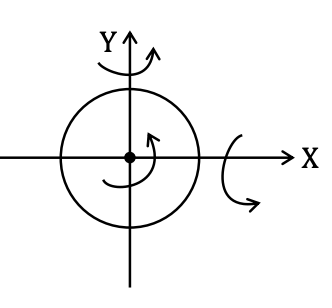Using perpendicular axis theorem:-

Iz = Ix + Iy … (1)

Given:-

Ix = 20 kg m2

Iy = 25 kg m2

Substitute values in equation (1),

I2 = 20 + 25

I2 = 45 kg m2

Question 15: Two wires A and B are stretched by the same load. If the area of cross-section of wire ‘A’ is double that of ‘B’, then the stress on ‘B’ is

1. a. Equal to that on A
2. b. Twice that on A
3. c. Half that on A
4. d. Four times that on A

Solution:

Stress = F / A

Stress A / Stress B = (F / AA) / (F / AB) = AB / AA

Given:-

Area of a cross-section of wire ‘A’ is double that of ‘B’

∴ AA = 2AB

Stress A / Stress B = AB / 2AB = 1 / 2

∴ Stress B = twice on stress ‘A’

Question 16: The magnitude of point charge due to which the electric field 30 cm away has the magnitude 2 NC–1 will be

1. a. 2 × 10–11C
2. b. 3 × 10–11 C
3. c. 5 × 10–11C
4. d. 9 × 10–11C

Solution:

The magnitude of the electric field is 2 N / C.

Distance r = 30 cm ⇒ 30 × 10-2 m

Apply formula of E.F.

E = Kq / r2

2 = [9 × 109 × q] / [30 × 10-2]2

q = [2 × (30 × 10-2)2] / [9 × 109]

q = (2 × (900 × 10-4)) / (9 × 109)

After solving

Q = 2 × 10-11 C

Question 17: A mass of 1 kg carrying a charge of 2 C is accelerated through a potential of 1 V. The velocity acquired by it is

1. a. √2 ms–1
2. b. 2 ms–1
3. c. 1 / √2 ms–1
4. d. 1 / 2 ms-1

Solution:

Given:-
m = 1 kg

q = 2C

Potential (V) = 1 V

Electrostatic Potential Energy = K.E.

q × V = ½ MV2

2 × 1 = ½ × 1 × V2

V = 2 m / s

Question 18: The force of repulsion between two identical positive charges when kept, with a separation ‘r’ in the air is ‘F’. Half the gap between the two charges is filled by a dielectric slab of dielectric constant = 4. Then, the new force of repulsion between those two charges becomes

1. a. F / 3
2. b. F / 2
3. c. F / 4
4. d. 4F / 9

Solution:

Case 1:

Force (f) = kq1q2 / r2 ----- (1)

F = [1 / (4𝝿𝜺0)] [q1q2 / r2]

Case 2:

New distance after a dielectric slab is inserted between 2 changes,

dnew = [(r / 2) + (r / 2) * √4]

⇒ (r / 2) + r ⇒ 3r / 2

Thus,

New force [F1] = [1 / (4𝝿𝜺0)] [q1q2 / (3r / 2)2] ---- (2)

Equation (ii) dividing by equation (i)

F1 / F = {[1 / (4𝝿𝜺0)] [q1q2 / (9r2 / 4)]} / {[1 / (4𝝿𝜺0)] [q1q2 / r2]}

After solving,

F’ = 4F / 9

Question 19: For the arrangement of capacitors as shown in the circuit, the effective capacitance between point A and B is (capacitance of each capacitor is 4μF)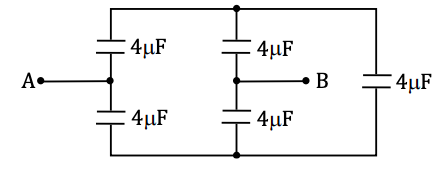1. a. 4 μF
2. b. 2 μF
3. c. 1 μF
4. d. 8 μF

Solution: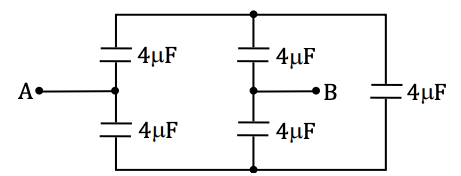From the balanced Wheatstone bridge,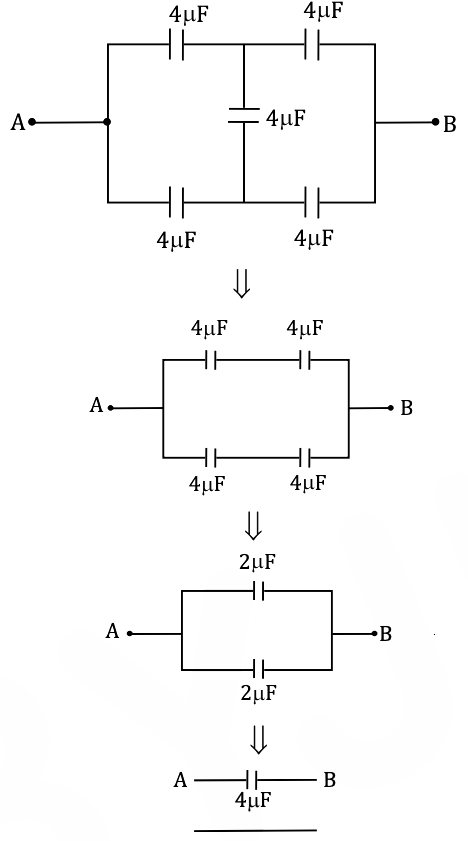Question 20: The work done to move a charge on an equipotential surface is

1. a. Infinity
2. b. Less than 1
3. c. Greater than 1
4. d. Zero

Solution:

An equipotential surface is one in which all the points are at the same electric potential, so the work done to move a charge on an equipotential surface is zero. According to the formula,

dw = q.dv

Question 21: Two capacitors of 3 μF and 6 μF are connected in series and a potential difference of 900 V is applied across the combination. They are then disconnected and reconnected in parallel. The potential difference across the combination is

1. a. Zero
2. b. 100 V
3. c. 200 V
4. d. 400 V

Solution: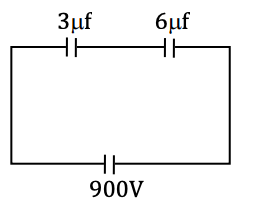C1 = 3μF, C2 = 6μF, When there capacitor disconnected

The series combination charge on & reconnected in parallel the

Both capacitors is same charge & P.D. is same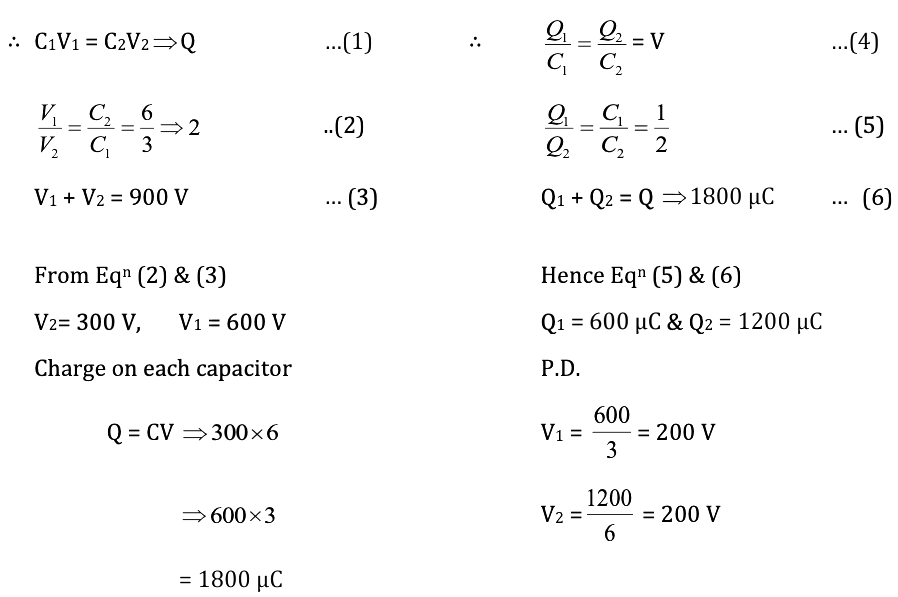Question 22: Ohm’s Law is applicable to

1. a. Diode
2. b. Transistor
3. c. Electrolyte
4. d. Conductor

Solution:

Ohm’s law is applicable only to conductors.

Question 23: If the last band on the carbon resistor is absent, then the tolerance is

1. a. 5 %
2. b. 20 %
3. c. 10 %
4. d. 15 %

Solution:

If the last bond on the carbon resistor is absent, there is no tolerance band, it is 20%.

Question 24: The effective resistance between P and Q for the following network is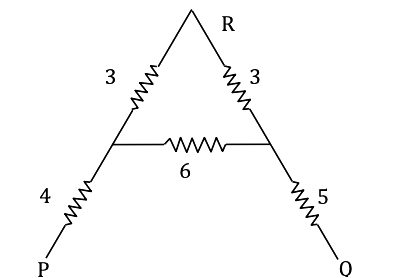1. a. (1 / 12) Ω
2. b. 21Ω
3. c. 12Ω
4. d. (1 / 21)Ω

Solution: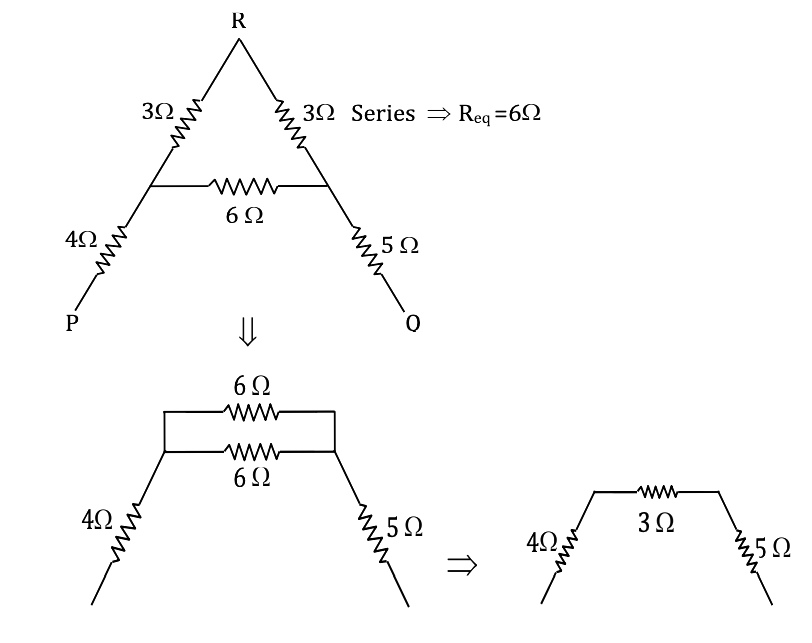Req. = 4 + 3 + 5 = 12Ω

Question 25: Five identical resistors each of resistance R = 1500 are connected to a 300 V battery as shown in the circuit. The reading of the ideal ammeter A is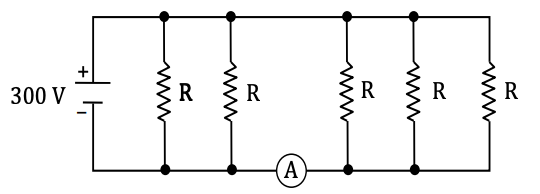1. a. (1 / 5) A
2. b. (3 / 5) A
3. c. (2 / 5) A
4. d. (4 / 5) A

Solution: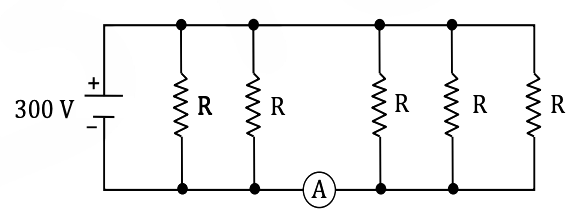Using formula V = IR

I = V / R

All resistances are in parallel.

∴ I = V / (R / 5) = [300 * 5] / R

Given:- R ⇒ 1500

Current through (A) = (3 / 5) A.

Question 26: Two cells of internal resistances r1 and r2 and of the same emf are connected in series, across a resistor of resistance R. If the terminal potential difference across the cells of internal resistance r1 is zero, then the value of R is

1. a. R = 2 (r1 + r2)
2. b. R = r2 – r1
3. c. R = r1 – r2
4. d. R = 2 (r1 – r2)

Solution: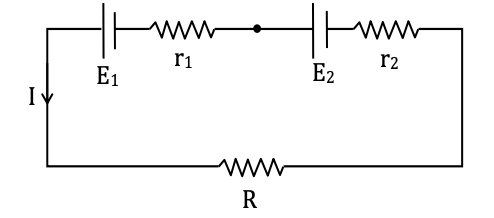According to Ohm’s law V = IR

E + E = 2I (r1 + r2 + R)

I = (2E) / (R + r1 + r2)

The terminal potential difference across the cells of internal resistance r1 = 0.

V = E - Ir1

0 = E1 – Ir

I = E1 / r1

(2E) / (R + r1 + r2) = E / r1

2r1 = R + r1 + r2

R = r1 - r2

Question 27: The I – V graphs for two different electrical appliances P and Q are shown in the diagram. If RP and RQ be the resistances of the devices, then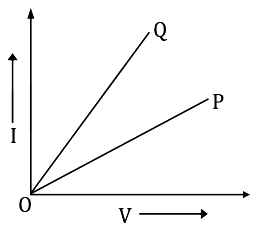1. a. RP = RQ
2. b. RP > RQ
3. c. RP < RQ
4. d. RP = RQ / 2

Solution: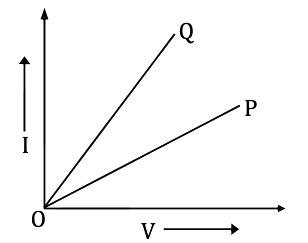The slope of this graph is the reciprocal of resistance.

Q (slope) > P (slope)

(1 / RQ) > (1 / RP)

RP > RQ

Question 28: The correct Biot-Savart law in vector form is

1. a. $\ d\vec{B}=\frac{\mu _{0}}{4\pi}\frac{I(d\vec{l}\times \vec{r})}{r^{2}}\\$
2. b. $\ d\vec{B}=\frac{\mu _{0}}{4\pi}\frac{I(d\vec{l}\times \vec{r})}{r^{3}}\\$
3. c. $\ d\vec{B}=\frac{\mu _{0}}{4\pi}\frac{I(d\vec{l})}{r^{2}}\\$
4. d. $\ d\vec{B}=\frac{\mu _{0}}{4\pi}\frac{I(d\vec{l})}{r^{3}}\\$

Solution:

Biot–Savart law:-

dB = (μ0 / 4π) (Idl sin θ / r2)

Vector form:-

Now we know,

$\hat{r}=\frac{\vec{r}}{r}\\ \vec{dB}=\frac{\mu _{0}}{4\pi}\frac{Idlsin\Theta}{r^{2}}\times \hat{r}\\ \vec{dB}=\frac{\mu _{0}}{4\pi}\frac{I(d\vec{l}\times \vec{r})}{r^{3}}$

Question 29: An electron is moving in a circle of radius r in a uniform magnetic field B. Suddenly, the field is reduced to B / 2. The radius of the circular path now becomes

1. a. r / 2
2. b. 2r
3. c. r / 4
4. d. 4r

Solution:

R = mV / qB

Thus, r1 B1 = r2 B2

r . B = r1 . [B / 2]

r1 = 2r

Question 30: A charge q is accelerated through a potential difference V. It is then passed normally through a uniform magnetic field, where it moves in a circle of radius r. The potential difference required to move it in a circle of radius 2r is

1. a. 2 V
2. b. 4 V
3. c. 1 V
4. d. 3 V

Solution:

r = mV / qB

For acceleration energy,

ev = (1 / 2) mv2

v2 = 2eV / m

v = √2eV / m

r ∝ √V

V ∝ r2

So,

V2 / V1 = (r2 / r1)2

After solving

V2 = 4V1

Question 31: A cyclotron’s oscillator frequency is 10 MHz and the operating magnetic field is 0.66 T. If the radius of its dees is 60 cm, then the kinetic energy of the proton beam produced by the accelerator is

1. a. 9 MeV
2. b. 10 MeV
3. c. 7 MeV
4. d. 11 MeV

Solution:

Frequency, v = 10 MHz ⇒ 107 Hz

Magnetic field:-

B = 2πmv / q

∴ (2 × (22 / 7) × 1.6 × 10-27 × 107) / (1.6 × 10-19) ⇒ 10.4876 / 1.6

After solving

B = 0.656 T

Now energy:-

Emax = B2q2R2 / M

= [(0.656)2 × (1.67 × 10-27)2 × (60 × 10-2)2] / [2 × 1.67 × 10-27]

After solving, = 7 MeV

Question 32: Needles N1, N2 and N3 are made of a ferromagnetic, a paramagnetic and a diamagnetic substance respectively. A magnet, when brought close to them, will

1. a. Attract all three of them
2. b. Attract N1 strongly, N2 weakly and repel N3 weakly
3. c. Attract N1 strongly but repel N2 and N3 weakly
4. d. Attract N1 and N2 strongly but repel N3

Solution: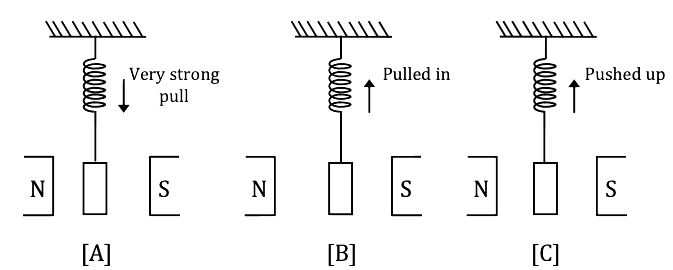a) These are strongly attracted in an external magnetic field [In ferromagnetic substance]

b) These are feebly attracted in an external magnetic field (in Paramagnetic substance).

c) These are repelled in an external magnetic field. (In diamagnetic Substance)

So,

A magnet will attract N1 strongly, N2 weakly and repel N3 weakly.

Question 33: The strength of the Earth’s magnetic field is

1. a. Constant everywhere
2. b. Zero everywhere
3. c. Having very high value
4. d. Varying from place to place on the Earth’s surface

Solution:

A magnetic field extends infinitely. The strength of the earth’s magnetic field is not constant. It varies from one place to another place on the surface of the earth.

Question 34: A jet plane with a wing-span of 25 m is travelling horizontally towards the east with a speed of 3600 km/hour. If the Earth’s magnetic field at the location is 4 × 10–4 T and the angle of dip is 30°, then, the potential difference between the ends of the wing is

1. a. 4 V
2. b. 5 V
3. c. 2 V
4. d. 2.5 V

Solution:

Length (l) = 25 m

Speed (V) = 3600 KM/hour

= 3600 × (5 / 18)

= 200 × 5

V = 1000 m/s

Magnetic field B = 4 × 10–4 T

θ = 30°

Vertical component of earth (BV)

BV = B . sin θ

Ε = (BV) × l × V ⇒ B . sin θ × l × V

= 4 × 10-4 × ½ × 25 × 1000

After solving,

E = 5 Volt

Question 35: Which of the following represents the variation of inductive reactance (XL) with the frequency of voltage source (v)?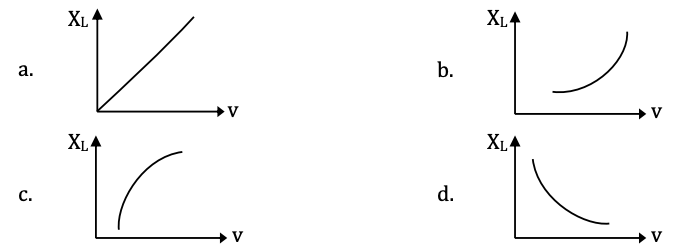Solution: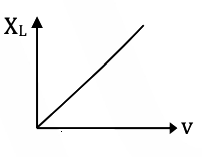We know that

Inductive reactance

XL = ωL

Frequency = v

XL = 2πvL

XL = 2πL × v

This equation can be compared to the equation of straight line Y = m × C.

Question 36: The magnetic flux linked with a coil varies as Φ = 3t2 + 4t + 9. The magnitude of the emf induced at t = 2 seconds is

1. a. 8 V
2. b. 16 V
3. c. 32 V
4. d. 64 V

Solution:

e = dΦ / dt

Φ = 3t2 + 4t + 9

So,

e = 6t + 4

t = 2 sec.

e = 6 × 2 + 4

e = 16 v

Question 37: A 100 W bulb is connected to an AC source of 220 V, 50 Hz. Then the current flowing through the bulb is

1. a. [5 / 11] A
2. b. [1 / 2] A
3. c. 2 A
4. d. [3 / 4] A

Solution:

Power (P) = 100 w

V = 220

We know that

P = VI

I = P / V ⇒ 100 / 220

I = [5 / 11] Amp

Question 38: In the series LCR circuit, the power dissipation is through

1. a. R
2. b. L
3. c. C
4. d. Both L and C

Solution:

The formula used power dissipated in an LCR circuit

P = Vrms. Irms cos Φ

Question 39: In Karnataka, the normal domestic power supply AC is 220 V, 50 Hz. Here 220 V and 50 Hz refer to

1. a. Peak value of voltage and frequency
2. b. Rms value of voltage and frequency
3. c. Mean value of voltage and frequency
4. d. Peak value of voltage and angular frequency

Solution:

irms = V0 / √2

rms value of voltage & frequency

Question 40: A step-up transformer operates on a 230 V line and l loads current of 2 A. The ratio of primary and secondary windings is 1:25. Then the current in the primary is

1. a. 25 A
2. b. 50 A
3. c. 15 A
4. d. 12.5 A

Solution:

No. of primary winding = 1

No. of secondary winding = 25

Used formula

NP / NS = IS / IP

So,

IP = IS × (NS / NP)

Substitute values

IP = 2 × (25 / 1)

IP = 50A

Question 41: The number of photons falling per second on a completely darkened plate to produce a force of 6.62 × 10–5 N is ‘n’. If the wavelength of the light falling is 5 × 10–7 m, then n = _____× 1022. (h = 6.62 × 10–34 J–s)

1. a. 1
2. b. 5
3. c. 0.2
4. d. 3.3

Solution:

Wavelength (λ) = 5 × 10-7

Force (F) = 6.62 × 10-5 N

h = 6.62 × 10-34 J.S

Used formula

P = (n / t) . (hc / λ)

Fc = (n / t) . (hc / λ)

(n / t) = (Fλ / h)

= (6.62 × 10-5 × 5 × 10-7) / (6.62 × 10-34)

= 5 × 1022 V

Question 42: An object is placed at the principal focus of a convex mirror. The image will be at

1. a. Centre of curvature
2. b. Principal focus
3. c. Infinity
4. d. No image will be formed

Solution: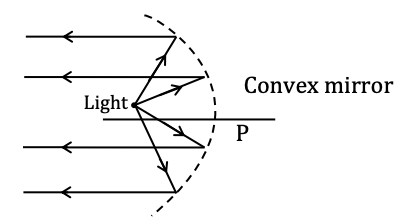When the object is placed at the focus the image is formed at infinity & highly emerged.

Question 43: An object is placed at a distance of 20 cm from the pole of a concave mirror of focal length 10 cm. The distance of the image formed is

1. a. + 20 cm
2. b. + 10 cm
3. c. –20 cm
4. d. – 10 cm

Solution:

Apply mirror formula

u = - 20 cm

F = 10 cm

(1 / f) = (1 / v) + (1 / u)

Question 44: A candle placed 25 cm from a lens forms an image on the screen placed 75 cm on the other side of the lens. The focal length and type of the lens should be

1. a. + 18.75 cm and convex lens
2. b. – 18.75 cm and concave lens
3. c. + 20.25 cm and convex lens
4. d. –20.25 cm and concave lens

Solution:

Using mirror formula

(1 / f) = (1 / v) + (1 / u)

Given:-

Object initial distance (u) = – 25 cm

Image distance (v) = +75 cm

Substitute values

(1 / f) = (1 / 75) - (1 / -25)

(1 / f) = (1 / 75) + (1 / 25) ⇒ (1 / f) = (1 + 3) / 75 ⇒ (1 / f) = 4 / 75

After solving f = + 18.75 cm and convex lens

Question 45: A plane wavefront of wavelength λ is incident on a single slit of width a. The angular width of the principal maximum is

1. a. λ / a
2. b. 2λ / a
3. c. a / λ
4. d. a / 2λ

Solution:

Fringe width ⇒ β = λD / d

Angular width θ ⇒ β / D

∴ θ = (λD / dD)

θ = λ / d
d = a (given)

Angular width θ = λ / a

Question 46: In a Fraunhofer diffraction at a single slit, if yellow light illuminating the slit is replaced by blue light, then diffraction bands

1. a. Remain unchanged
2. b. Become wider
3. c. Disappear
4. d. Become narrower

Solution:

When the blue light is used instead of yellow, decreases & hence, diffraction bands become narrower.

Question 47: In Young’s double-slit experiment, two wavelengths λ1 = 780 nm and λ2 = 520 nm are used to obtain interference fringes. If the nth bright band due to λ1 coincides with (n + 1)th bright band due to λ2, then the value of n is

1. a. 4
2. b. 3
3. c. 2
4. d. 6

Solution:

λ1 = 780 nm

λ2 = 520 nm

We know that,

The distance from the central maxima to nth bright band

yn = nλD / d

1D / d = (n + 1) (λ2D / d)

n1λ1 = (n + 1) λ2

(n + 1) / n = (λ1 / λ2) ⇒ 780 / 520

(n + 1) / n = 78 / 52

52 n + 52 = 78 n

26 n = 52

n = 2

Question 48: In Young’s double-slit experiment, slits are separated by 2 mm and the screen is placed at a distance of 1.2 m from the slits. Light consisting of two wavelengths 6500 Å and 5200 Å is used to obtain interference fringes. Then the separation between the fourth bright fringes of two different patterns produced by the two wavelengths is

1. a. 0.312 mm
2. b. 0.123 mm
3. c. 0.213 mm
4. d. 0.412 mm

Solution:

λ1 = 6500 Å

γ1 = 4λ1D / d

λ2 = 5200 Å

γ2 = 4λ2D / d

γ1 - γ2 = (4D / d) (λ1 - λ2)

= {[4 × 1.2] / [2 × 16-3]} (6500 - 5200)

= [4 × 1.2 × 1300 × 10-10] / [2 × 10-3]

After solving = 0.312 mm.

Question 49: The maximum kinetic energy of emitted photoelectrons depends on

1. a. Intensity of incident radiation
2. b. Frequency of incident radiation
3. c. Speed of incident radiation
4. d. Number of photons in the incident radiation

Solution:

By Einstein’s photoelectric equation

K.E. of the photoelectron is

½ mv2 = hv

K.E. of emitted photoelectrons depends on the frequency of incident radiations.

Question 50: A proton and an α particle are accelerated through the same potential difference V. The ratio of their de Broglie wavelengths is

1. a. √2
2. b. 2 √2
3. c. √3
4. d. 2 √3

Solution:

De-Broglie wavelength

λ = h / mv [∴P = mv]

De-Broglie wavelength of a proton:-

Mass = m1

λ1 = h / √2m1k [∴P = √2mk]

[k = qv]

∴ λ1 = h / √2m1kv ----- (1)

For an α-particle

Mass = m2

Charge = q0

∴ λ2 = h / √2m2q0v

For α – particle

24He ⇒ q0 = 2q

M2 = 4m1

∴ λ2 = h / √[2 × 4m1 × 2q× v] ------ (2)

Equation (1) and (2),

1 / λ2] = h / √2m1qv = √[2 × 4m1 × 2q× v] / h

After solving,

1 / λ2] = 2√2

Question 51: The total energy of an electron revolving in the second orbit of the hydrogen atom is

1. a. – 13.6 eV
2. b. –1.51 eV
3. c. –3.4 eV
4. d. Zero

Solution:

Energy:-

En = – 13.62 / n2

For hydrogen atom

Z = 1; n = 2

En = [– 13.62 × 12] / 22

= – 13.6 / 4

= – 3.4eV

Question 52: The period of revolution of an electron in the ground state of the hydrogen atom is T. The period of revolution of the electron in the first excited state is

1. a. 2 T
2. b. 4 T
3. c. 6 T
4. d. 8 T

Solution:

Time period (T) = (2πr / v)

= r × (n2 / z)

So,

T × (n3 / z2)

First excited state, n = 2

(T2 / T1) = (n2 / n1)3 = (2 / 1)3

T2 = 8T

Question 53: The energy equivalent to a substance of mass 1 g is

1. a. 18 × 1013 J
2. b. 9 × 1013 J
3. c. 18 × 106 J
4. d. 9 × 106 J

Solution:

M = 1g ⇒ 10-3 kg

Using Einstein’s equation:-

E = mc2

= 10-3 × (3 × 108)2

= 10-3 × 9× 1016

E = 9 × 1013

Question 54: The half-life of tritium is 12.5 years. What mass of tritium of initial mass 64 mg will remain undecayed after 50 years?

1. a. 32 mg
2. b. 8 mg
3. c. 16 mg
4. d. 4 mg

Solution:

Un-decayed

(N / N0) = (1 / 2x)

X = 50 / 12.5 = 4

N = N0 / 16 = 64 / 16 = 4mg

Question 55: In a CE amplifier, the input ac signal to be amplified is applied across

1. a. Forward biased emitter-base junction
2. b. Reverse biased collector-base junction
3. c. Reverse biased emitter-base junction
4. d. Forward biased collector-base junction

Solution: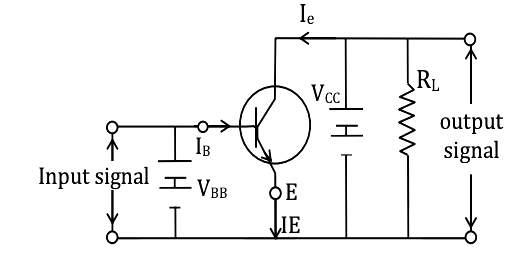The input ac signal to be amplified is applied across the forward-biased emitter-base junction.

Question 56: If A = 1 and B = 0, then in terms of Boolean algebra, $\bar{A}$ + B =

1. a. B
2. b. $\bar{B}$
3. c. A
4. d. $\bar{A}$

Solution:

A = 1, B = 0

$\\ \bar{A}+B=\bar{I}+0=0+0=0\\ \bar{A}+B=B$

Question 57: The density of an electron-hole pair in pure germanium is 3 × 1016 m–3 at room temperature. On doping with aluminium, the hole density increases to 4.5 × 1022 m–3. Now the electron density (in m–3) in doped germanium will be

1. a. 1 × 1010
2. b. 2 × 1010
3. c. 0.5 × 1010
4. d. 4 × 1010

Solution:

n1 = 3 × 1016 m-3

nn = 4.5 × 1022 m-3

n12 = nnne

ne = n12 / nn

= [3 × 1016]2 / [4.5 × 1022]

ne = [9 × 1032] / [4.5 × 1022]

ne = 2 × 1010

Question 58: The dc common-emitter current gain of a n-p-n transistor is 50. The potential difference applied across the collector and emitter of a transistor used in CE configuration is, VCE = 2 V. If the collector resistance, RC = 4Ω k, the base current (IB) and the collector current (IC) are

1. a. IB = 10 μA, IC = 0.5 mA
2. b. IB = 0.5 μA , IC = 10 mA
3. c. IB = 5 μA, IC = 1 mA
4. d. IB = 1 μA, IC = 0.5 mA

Solution:

IC = VCE / RC

Given;-

VCE = 2V

RC = 4 K

Substituting values

IC = 2 / [4 × 103] = 0.5 × 10-3 A

IC = 0.5 mA

β = IC / IB ⇒ IB = IC / β

= [0.5 × 10-3] / 50 = 10-5 A

IB = 10μA

Question 59: The radius of the Earth is 6400 km. If the height of an antenna is 500 m, then its range is

1. a. 800 km
2. b. 100 km
3. c. 80 km
4. d. 10 km

Solution:

Range = √2Rh

= √2 × 6400 × 103 × 500

= 80 × 103

= 80 cm

Question 60: A space station is at a height equal to the radius of the Earth. If ‘VE’ is the escape velocity on the surface of the Earth, the same on the space station is ______ times VE.

1. a. 1 / 2
2. b. 1 / 4
3. c. 1 / √2
4. d. 1 / √3

Solution:

[– GMm / (R + R)] + (1 / 2) mv2 = 0

(1 / 2) mv2 = [– GMm / 2R]

V’ = √GM / R ⇒ √gR

VE = √2gR

VE / V’ = √(2gR / gR)

V’ = VE × (1 / √2)

### KCET 2018 Physics Question Paper with Solutions